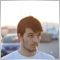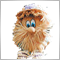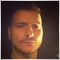# Partial close tickets separately1820

Hi there, I wrote a code to partial close orders else in profit or los at specified distance or current profit/loss by equity.

The problem is that expert's currently partially closing last ticket not each one separately, I mean for example:

First order: 0,03, second order 0,05, partial close 1/3.

Instead of doing this: Close 0,01/0,03 & 0,02/0,05. It's closing this way: 0,03/0,05 from the first ticket.

This is 1/3 from the total volume from the first ticket, not each one independent. How to solve?

```      for(int j=PositionsTotal()-1;j>=0;j--){
ulong PTicket=PositionGetTicket(j);
if(PositionSelectByTicket(PTicket)){
PP=PositionGetDouble(POSITION_PROFIT);
if(PP>=0){P+=PP;}else if(PP<0){L+=PP;}
PCP1=NormalizeDouble(PV*(PCloseT1/100),2);
PCP2=NormalizeDouble(PV*(PCloseT2/100),2);
double PSL=PositionGetDouble(POSITION_SL);
double PTP=PositionGetDouble(POSITION_TP);
double AE=AccountInfoDouble(ACCOUNT_EQUITY);
double AB=AccountInfoDouble(ACCOUNT_BALANCE);
PPC=PositionGetDouble(POSITION_PRICE_CURRENT);
if(PositionGetString(POSITION_SYMBOL)==SName){
double SPoint=SymbolInfoDouble(SName,SYMBOL_POINT);
//+---------------------------------------------------!
if(AE>AB*((1+GTarget2/2)/100)||P>AB*(RControl/100)){
if(PSL<DBEL&&PPC>DBEL+(PCloseT1*10)*SPoint){ Long++;
Print(SName," PositionClosePartial at GTarget3 error ",trade.ResultRetcode());
return;
}
Print(SName," PositionModify SL to Breakeven point error ",trade.ResultRetcode());
return;
}
else{Alert(Long," long positions partial closed by ",PCloseT2,"% on ",SName," at GTarget3.");}
}
}```Moderator
220238

David Diez :

Hi there, I wrote a code to partial close orders else in profit or los at specified distance or current profit/loss by equity.

The problem is that expert's currently partially closing last ticket not each one separately, I mean for example:

First order: 0,03, second order 0,05, partial close 1/3 .

Instead of doing this: Close 0,01/0,03 & 0,02/0,05 . It's closing this way: 0,03/0,05 from the first ticket .

This is 1/3 from the total volume from the first ticket, not each one independent. How to solve?

Use the stylizer and you won’t miss the brackets:

```   for(int j=PositionsTotal()-1; j>=0; j--)
{
ulong PTicket=PositionGetTicket(j);
if(PositionSelectByTicket(PTicket))
{
PP=PositionGetDouble(POSITION_PROFIT);
if(PP>=0)
{
P+=PP;
}
else
if(PP<0)
{
L+=PP;
}
PCP1=NormalizeDouble(PV*(PCloseT1/100),2);
PCP2=NormalizeDouble(PV*(PCloseT2/100),2);
double PSL=PositionGetDouble(POSITION_SL);
double PTP=PositionGetDouble(POSITION_TP);
double AE=AccountInfoDouble(ACCOUNT_EQUITY);
double AB=AccountInfoDouble(ACCOUNT_BALANCE);
PPC=PositionGetDouble(POSITION_PRICE_CURRENT);
if(PositionGetString(POSITION_SYMBOL)==SName)
{
double SPoint=SymbolInfoDouble(SName,SYMBOL_POINT);
{
//+---------------------------------------------------!
if(AE>AB*((1+GTarget2/2)/100)||P>AB*(RControl/100))
{
if(PSL<DBEL&&PPC>DBEL+(PCloseT1*10)*SPoint)
{
Long++;
{
Print(SName," PositionClosePartial at GTarget3 error ",trade.ResultRetcode());
return;
}
else
{
Print(SName," PositionModify SL to Breakeven point error ",trade.ResultRetcode());
return;
}
else
{
Alert(Long," long positions partial closed by ",PCloseT2,"% on ",SName," at GTarget3.");
}
}
}
}
}
}
}```Moderator
220238

I also recommend doing this:

create an array (structure) in which to record the TICKET and volume WHICH MUST BE PARTially closed.

At the first pass through the position cycle, fill the array.

And only the field of this process the array.Moderator
220238

Code:

```//+------------------------------------------------------------------+
//|                                                      Test_en.mq5 |
//+------------------------------------------------------------------+
//---
CPositionInfo  m_position;                   // object of CPositionInfo class
CSymbolInfo    m_symbol;                     // object of CSymbolInfo class
#property script_show_inputs
//--- input parameters
input ulong    InpMagic             = 200;         // Magic number
//+------------------------------------------------------------------+
//| Script program start function                                    |
//+------------------------------------------------------------------+
void OnStart()
{
if(!m_symbol.Name(Symbol())) // sets symbol name
{
Print(__FILE__," ",__FUNCTION__,", ERROR: CSymbolInfo.Name");
return;
}
//---
struct SPartial
{
ulong          ticket;          // ticket
double         partial_volume;  // partial volume
};
SPartial arr_partial[];
for(int i=PositionsTotal()-1; i>=0; i--) // returns the number of current positions
if(m_position.SelectByIndex(i)) // selects the position by index for further access to its properties
if(m_position.Symbol()==m_symbol.Name() && m_position.Magic()==InpMagic)
{
if(...)
{
int size=ArraySize(arr_partial);
ArrayResize(arr_partial,size+1);
arr_partial[size].ticket=m_position.Ticket();
arr_partial[size].partial_volume=m_position.Volume()/3.0;
}
}
//---
int size=ArraySize(arr_partial);
for(int i=size-1; i>=0; i--)
{
}
//---
ArrayFree(arr_partial);
}
//+------------------------------------------------------------------+
```
Files:
Test_en.mq5 5 kb24345

Does the Partial remaining order get a new ticket in MT5 as in MT4 ?Moderator
220238

Lorentzos Roussos :
Does the Partial remaining order get a new ticket in MT5 as in MT4 ?

Example:

```//+------------------------------------------------------------------+
//|                                                      Test_en.mq5 |
//+------------------------------------------------------------------+
//---
CPositionInfo  m_position;                   // object of CPositionInfo class
CSymbolInfo    m_symbol;                     // object of CSymbolInfo class
#property script_show_inputs
//--- input parameters
input ulong    InpMagic             = 200;         // Magic number
//+------------------------------------------------------------------+
//| Script program start function                                    |
//+------------------------------------------------------------------+
void OnStart()
{
if(!m_symbol.Name(Symbol())) // sets symbol name
{
Print(__FILE__," ",__FUNCTION__,", ERROR: CSymbolInfo.Name");
return;
}
//---
struct SPartial
{
ulong          ticket;          // ticket
double         partial_volume;  // partial volume
};
SPartial arr_partial[];
Print("- Before partial closure -");
for(int i=PositionsTotal()-1; i>=0; i--) // returns the number of current positions
if(m_position.SelectByIndex(i)) // selects the position by index for further access to its properties
if(m_position.Symbol()==m_symbol.Name() && m_position.Magic()==InpMagic)
{
if(1==1)
{
Print("Ticket: ",IntegerToString(m_position.Ticket()),", volume: ",DoubleToString(m_position.Volume(),2));
int size=ArraySize(arr_partial);
ArrayResize(arr_partial,size+1);
arr_partial[size].ticket=m_position.Ticket();
arr_partial[size].partial_volume=m_position.Volume()/3.0;
}
}
//---
int size=ArraySize(arr_partial);
for(int i=size-1; i>=0; i--)
{
}
//---
ArrayFree(arr_partial);
//---
Print("- After partial closure -");
for(int i=PositionsTotal()-1; i>=0; i--) // returns the number of current positions
if(m_position.SelectByIndex(i)) // selects the position by index for further access to its properties
if(m_position.Symbol()==m_symbol.Name() && m_position.Magic()==InpMagic)
{
if(1==1)
{
Print("Ticket: ",IntegerToString(m_position.Ticket()),", volume: ",DoubleToString(m_position.Volume(),2));
}
}
}
//+------------------------------------------------------------------+
```

Result

```2019.10.23 15:38:32.199 Test_en (EURUSD,H1)     - Before partial closure -
2019.10.23 15:38:32.199 Test_en (EURUSD,H1)     Ticket: 469986986, volume: 0.03
2019.10.23 15:38:34.843 Test_en (EURUSD,H1)     - After partial closure -
2019.10.23 15:38:34.843 Test_en (EURUSD,H1)     Ticket: 469986986, volume: 0.02 ```

POSITION ticket Before partial closure == POSITION ticket after partial closure

Files:
Test_en.mq5 6 kb24345

Great ! Thanks for (taking) the time to write it .1820

Code:

Thank you mate, I know you're a classic from the CodeBase, but I'd prefer to make simpler things without the use of complex solutions as I understand arrays.

Know that in fact they're not so complex but even trying to make it as simpler as I can. So the problem is, there is a deal of two positions which its position volume is… total volume.

It seems that Symbol and OrderType conditions are merging each single ticket into a single one, so at this point I think it's needed a new loop or a void function call to make it fair.Moderator
220238

David Diez :

Thank you mate, I know you're a classic from the CodeBase, but I'd prefer to make simpler things without the use of complex solutions as I understand arrays.

Know that in fact they're not so complex but even trying to make it as simpler as I can. So the problem is, there is a deal of two positions which its position volume is… total volume.

It seems that Symbol and OrderType conditions are merging each single ticket into a single one, so at this point I think it's needed a new loop or a void function call to make it fair.

Above, I gave an example (a very simple example) in the form of a script (not an adviser).

In the EA, the algorithm is different.1820